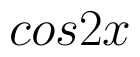Select Page

# 12 Science Maths CBSE Application of Integrals Solutions for MCQ in English

12 Science Maths CBSE Application of Integrals Solutions for MCQ in English to enable students to get Solutions in a narrative video format for the specific question.

Expert Teacher provides 12 Science Maths CBSE Application of Integrals Solutions for MCQ through Video Solutions in English language. This video solution will be useful for students to understand how to write an answer in exam in order to score more marks. This teacher uses a narrative style for a question from Application of Integrals not only to explain the proper method of answering question, but deriving right answer too.

Please find the question below and view the Solution in a narrative video format.

Question:

Solution Video in English:

## Similar Questions from CBSE, 12th Science, Maths, Application of Integrals

Question 1 : Find the area of the region enclosed by the parabolathe line y = x + 2. (View Answer Video)

Question 2 : Using integration, find the area of the region bounded by the line 2x + y = 4, 3x - 2y = 6 and x - 3y + 5 = 0. (View Answer Video)

Question 3 : Find the area of the region bounded by the curveand. (View Answer Video)

Question 4 : Find the area bounded by the curveand the line x = 4y - 2. (View Answer Video)

Question 5 : Find the area of the region in the first quadrant enclosed by x-axis, lineand the circle(View Answer Video)

### Application of Derivatives

Question 1 : The function f is a differentiable function and satisfies the functional equation f(x) + f(y) = f(x + y) – xy – 1for every pair x, y of real numbers. If f(1) = 1, then the number of integers n ≠ 1 for which f(n) = n is_______________. (View Answer Video)

Question 2 : The maximum value ofis (View Answer Video)

Question 3 : Find two positive numbers whose sum is 16 and the sum of whose cubes is minimum. (View Answer Video)

Question 4 : A cylindrical tank of radius 10 m is being filled with wheat at the rate of the 314 cubic meters per hour. Then the depth of the wheat is increasing at the rate of ___________. (View Answer Video)

Question 5 :

The total revenue in Rupees received from the sale of 'x' units of a product is given by :The marginal revenue, when x=15 is :

### Integrals

Question 1 : Find the integral of the function. (View Answer Video)

Question 2 : Evaluate :(View Answer Video)

Question 3 : Find :(View Answer Video)

Question 4 : Write an antiderivative for the function,using the method of inspection. (View Answer Video)

Question 5 : Find :(View Answer Video)

### Continuity and Differentiability

Question 1 : Differentiate the functionwith respect to x. (View Answer Video)

Question 2 : Differentiate the functionwith respect to x. (View Answer Video)

Question 3 : Findfor the function. (View Answer Video)

Question 4 : Differentiate the functionwith respect to x. (View Answer Video)

Question 5 : Findfor the function. (View Answer Video)